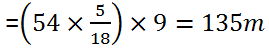# IBPS Online Exam – Time & Distance Problem with Solution

##### Share Via:

IBPS ONLINE EXAM – Time & Distance Problem with Solution – Here at IBPSCO we are trying to make you familiar with diffrent Section of ibpsonline exam and some sort of study material of ibps online exam. Hope this will helpful. comment below in case any confusion..

### Time & Distance Problem with Solution

1- How many minutes Raman will take to cover a distance of 400 meters if he runs at a speed of 20 km/hr ?
a. 2 mins
b. 1.5 mins
c. 1⅕ mins
d. 2.5 mins
e. None of these

Explanation :
⇒ Raman’s speed = 20 km/hr = 20 × 5/18 = 50/9 m/sec
⇒ 400 × 9/50 = 1⅕ mins

2- John travelled from his town to city.  John went to city by bicycle at the speed of 25 km/h and came back at the speed of 4 km/h. If John took 5 hours and 48 min to complete his journey, what is the distance between town and city ?

a. 15 km
b. 22 km

c. 20 km
d. 25 km

Explanation :
• Average speed of John = 2xy/x+y =  2 × 25 × 4 / 25 + 4= 200/29 km/h
• Distance traveled = Speed × Time = 200/29 × 29/5 = 40 Km
• Distance between city and town = 40/2 = 20 km
3- Speed of a train is 20 meters per second. It can cross a pole in 10 seconds. What is the length of train ?
a. 150 m
b. 250 m
c. 200 m
d. 300 m

Explanation :
⇒ Lenght of train = 20 × 10 = 200 meters

4-Ram walks at a speed of  12 km/h. Today the day was very hot so walked at ⅚ of his average speed. He arrived his school 10 minutes late. Find the usual time he takes to cover the distance between his school and home?

a. 40 mins
b. 45 mins
c. 50 mins
d. 60 mins

Explanation :
• If Ram is walking at ⅚  of his usual speed that means he is taking 6/5 of using time.
• 6/5 of usual time – usual time = 10 mins
• 1/5 of usual time = 10 mins
• Usual time = 50 mins

5-

A car running at 65 km/h takes one hour to cover a distance. If the speed is reduced by 15 km/hour then in how much time it will cover the distance  ?

a. 72 mins
b. 78 mins
c. 76 mins
d. None of these

Explanation :
⇒Reduced speed = 65-15 = 50 km/h
⇒ Now car will take 65/50 × 60 mins = 78 mins
6-In a 100 m race A runs at a speed of 1.66 m/s. If A gives a start of 4m to B and still beats him by 12 seconds. What is the speed of B  ?

a. 1 m/s
b. 1.33 m/s
c. 1.25 m/s

d. 1.5 m/s

Explanation :
• Time is taken by A to cover 100 meters = 60 seconds
• Since A gives a start of 4 seconds then time takes by B = 72 seconds
• B takes 72 seconds to cover 96 meters
• Speed of B = 96/72 = 1.33 m/s

7-

In a kilometer race, A beats B by 100 meters. B beats C by 100 meters. By how many meters does A beat C in the same race?

a. 200 meters
b. 180 meters
c. 190 meters
d. 210 meters

Explanation :
While A covers 1000 meters, B can cover 900 meters
While B cover 1000 meters, C can cover 900 meters
Lets assume that all three of them are running the same race. So when B runs 900 meters, C can run 900 × 9/10 =810
⇒ So A can beat C by 190 meters.

8-Without any stoppage a person travels a certain distance at an average speed of 42 km/hr and with stoppages he covers the same distance at an average speed of 28 km/hr. How many minutes per hour does he stop?
(a) 25 minutes

(b) 30 minutes
(c) 20 minutes
(d) None of these

Ans 8. (C)9-

A train passes through a telegraph post in 9 seconds moving at a speed of 54 km per hour. The length of the train is
(a) 135 meters
(c) 125 meters
(b) 145 meters
(d) None of these

Ans 9. (a)

Length of the train = Speed of the train  ✕ Time taken in crossing the post10-

A train 50 m long passes a platform 100 m long in 10 seconds. The speed of the train in m/sec. is
(a) 25
(b) 35
(c) 15
(d) None of these

Ans 10. (b)———————-
For all other Sarkari naukri job , result admit card  apart from banking you can Click here